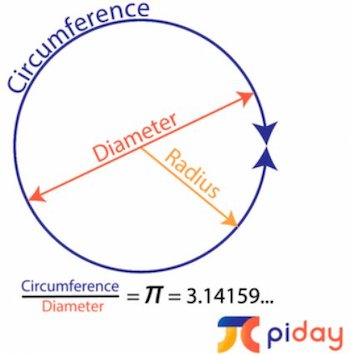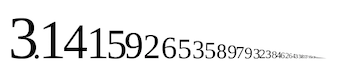# Happy Pi Day March 14Today, March 14, is Pi Day, celebrating the first three numbers of Pi (π) which are 3.14. The Greek letter π is merely the circumference or distance around a circle divided by its diameter or distance across the circle. Hence the formula C = π d or π = C/d. You may also remember this formula as C = 2 π r, because the diameter is equal to twice the radius (the radius is the distance from the center of the circle to the edge of the circle.)

According to exploratorium.edu, people have known about π for almost 4,000 years. The Babylonians, the Egyptians and even the Chinese knew of this ratio and attempted with rudimentary tools and calculators to find the exact number. Archimedes is credited with the first mathematical proof that π was 31/7 or 22/7 which is approximately 3.14.

It wasn’t until the 1700’s that the Greek letter π was used to denote this relationship between the circumference and the diameter.

Π is an irrational number. That means it goes on forever, never ends and doesn’t have a pattern. This is important because if you are trying to calculate something as exact as you can, you want to use the most accurate approximation of π.